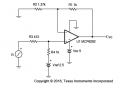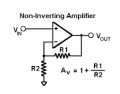# is there a way in which i can calculate resistor values instead of solving this complex equations

#### atferrari

Joined Jan 6, 2004
4,434

#### ericgibbs

Joined Jan 29, 2010
13,670
hi,
Set up a Spreadsheet, so that you enter the values and it does the calcs.
E

•MrSoftware

#### DickCappels

Joined Aug 21, 2008
7,748
What kind of amplifier is this, and where did you get that equation?

#### DickCappels

Joined Aug 21, 2008
7,748You can make this a lot simpler my looking at the circuit as two circuits and solve each one.

Simply find the values of R3 and R4 to give the offset voltage in the correct proportion to the input voltage. This is a simple resistive voltage divider problem.

After that use the formula below to calculate the resistor values needed to scale the offset signal at the noninverting input to the desired amplitude on the output.

The formula: The output of the amplifier is the voltage on the noninverting input times (1 + (R1/R2)).

/////////////////////////
Gain of a noninvertng amplifier:•ashokraj